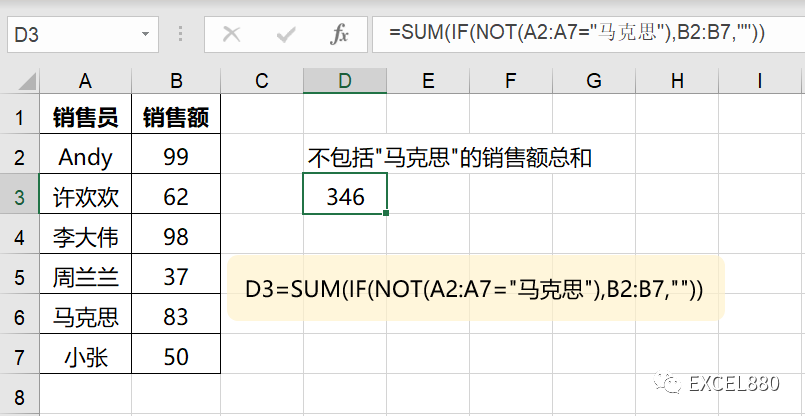# 10个Excel/WPS逻辑判断函数详解，再也不怕写条件了

205 次浏览

Excel有500多个函数，分为14个类别，可参考微软官网。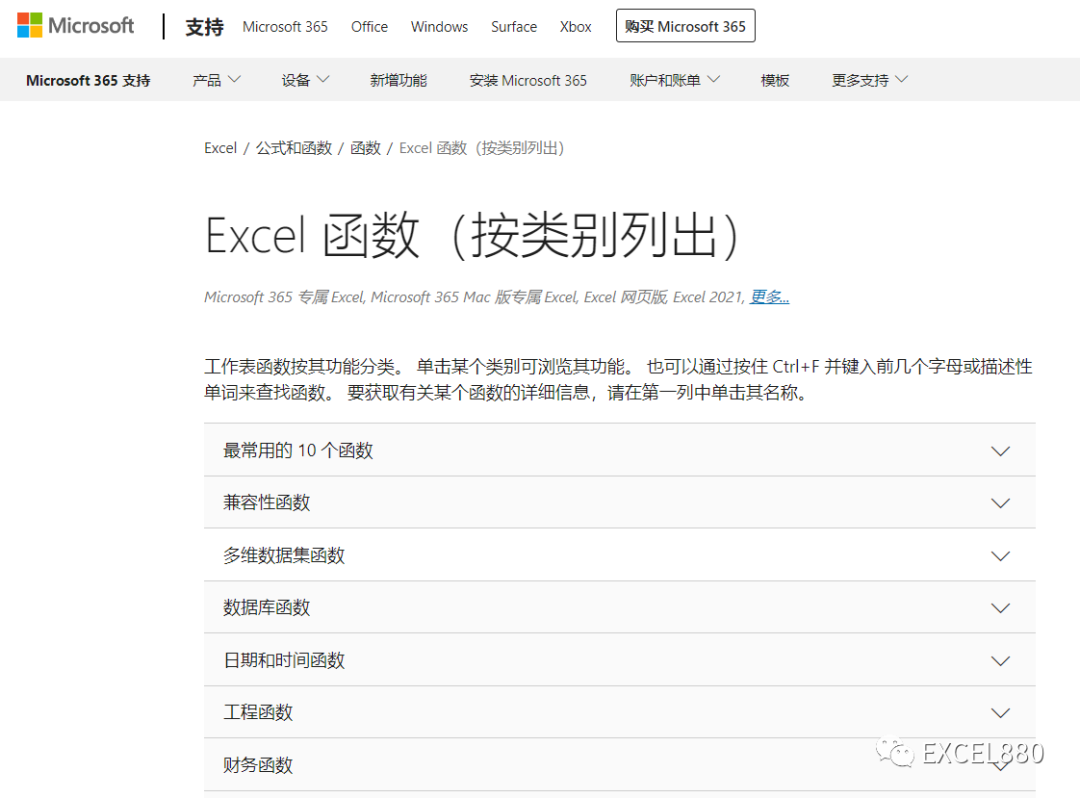TRUE,FALSE

IF

=IF(B2>=60,”及格”,”不及格”)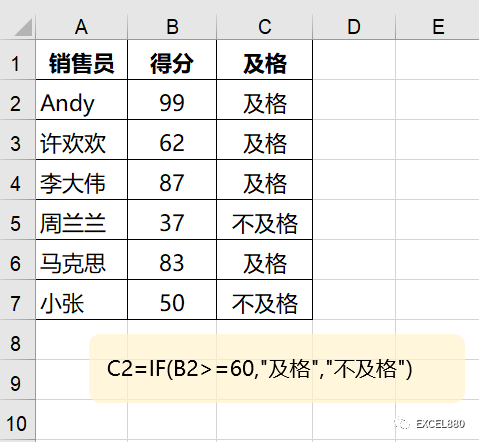IFS

=IFS(B2<50,”C”,B2<80,”B”,B2>=80,”A”)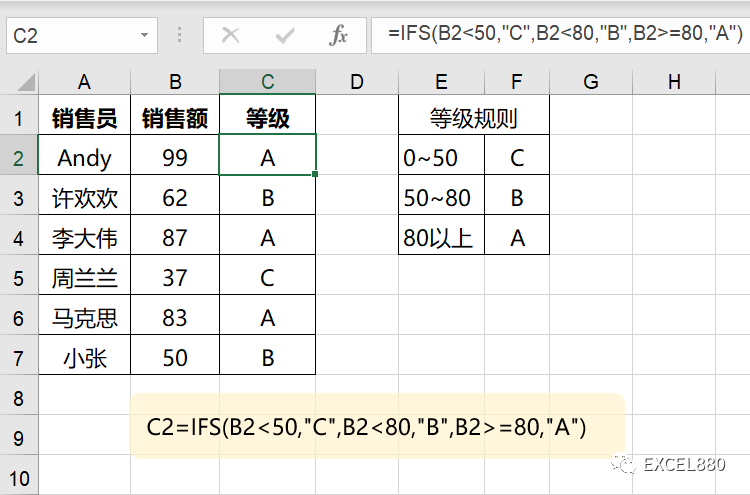IFERROR

=SUM(IFERROR(A1:D5,””))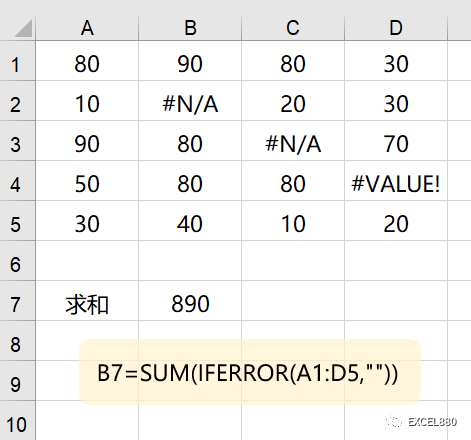LAMBDA

LAMBDA是M365版本推出的新函数，很意外，被归为逻辑函数。

=LAMBDA(数量,单价,数量*单价)(B2,C2)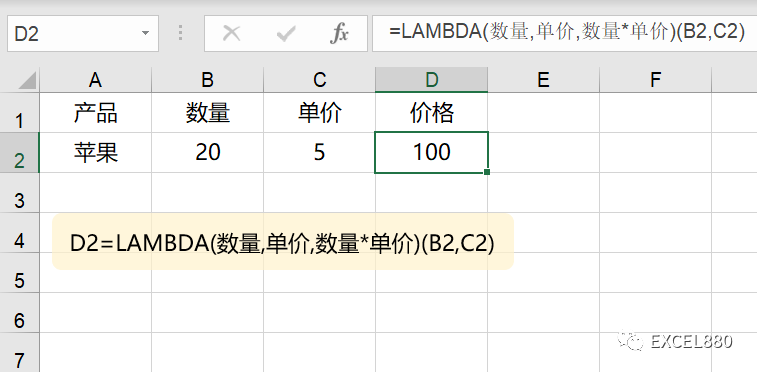LET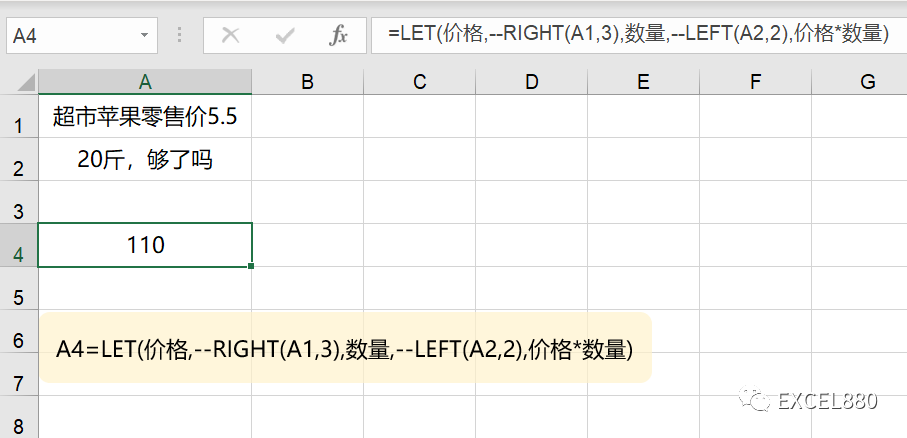AND

=IF(AND(B2>90,C2>90),”优”,””)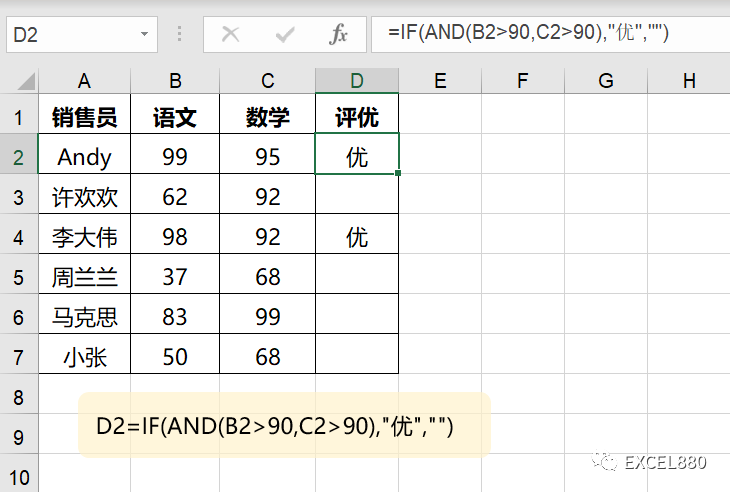OR

=IF(OR(B2>90,C2>90),”优”,””)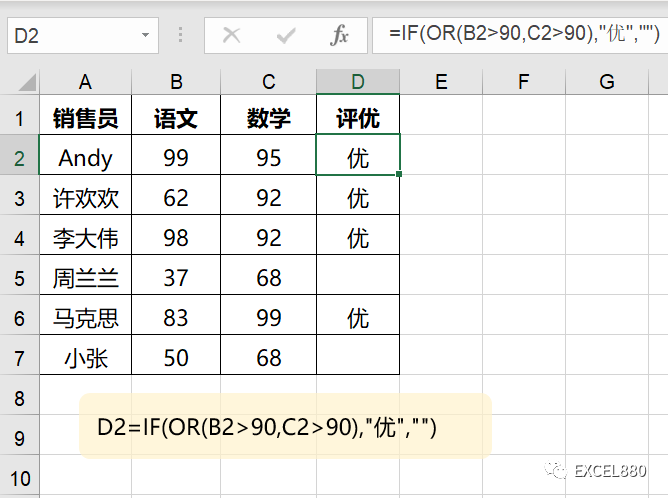NOT

=SUM(IF(NOT(A2:A7=”马克思”),B2:B7,””))